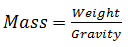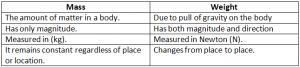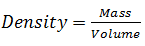Monday , June 24 2019
Home » Igcse Physics Revision Notes » Mass Weight And Density

Mass Weight And Density

Mass:

Mass is the measure of amount of substance in a body. (Irrespective of its shape, location and speed.

Mass depends on the number and composition of atoms and molecules.SI unit: kilogram (kg)

Remember: mass is a scalar quantity.

Mass can’t be changed by location, shape and speed of the body.

Weight:

Weight is a force and has direction.

Weight = Mass × Gravity

W = mg

SI unit: Newton (N)

The direction of the weight acts downwards towards the center of the earth.

It is sometimes also called gravitational force / gravity.

Mass And Weight Relation:

Mass is directly proportional to weight.

Thus if mass increases weight increases and vice versa if mass decreases weight also decreases.

How To Measure Mass And Weight:

In order to measure weight:

• Use electronic balance, spring balance and bathroom scales.
• These machines are calibrated to give readings in grams (g) or kilograms (kg).
• Weighing scales calibrated for use on earth can’t be used on the moon.

In order to measure mass:

• Use beam balance or calibrated electronic balance.

Difference Between Mass And Weight:Gravitaitonal Field:

The region around the earth where gravity is experienced is called the gravitational field.

Gravitational Field Strength:

It is defined as the gravitational force acting per unit mass on an object.

On earth gravitational field strength is about 10 Nkg-1

It means that 1 kg of mass experiences a force of 10 N kg-1

Gravitaional Force:

• All masses attract one another with this force.
• The larger the mass, the stronger the force.
• The smaller the distance between the mass the stronger the force.

Inertia:

Inertia of an object refers to the reluctance of the object to change its state of rest or motion.

Inertia of an object depends on its mass. An object with greater mass has greater inertia and vice versa an object with lower mass would have lower inertia.

In other words the larger the mass of an object, the harder it is to start moving , slow down, move faster or change direction.

Density:

Density is defined as  mass per unit volumeSI unit:  kgm-3  or  g/cm3

Finding density of a regular shaped object:

Use a beam balance to measure mass

Use a ruler to measure the sides and calculate volume using formula of the volume of shape.

Calculate density by using the formula:  Density = mass / volume

Finding density of an irregular shaped object:

Use a beam balance to measure mass

Lower the object into a half filled measuring cylinder gently and measure the volume by subtracting initial water level from final water level.Turning Effects Of Forces

Moments: The moment of a force is the product of the force and the perpendicular …

1.sakchyat parajuli

2.3.